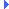FD.io VPP  v18.01-8-g0eacf49 Vector Packet Processing
math.h File ReferenceInclude dependency graph for math.h:This graph shows which files directly or indirectly include this file:

Go to the source code of this file.

## Macros

#define isnan(x)   __builtin_isnan(x)

#define isinf(x)   __builtin_isinf(x)

## Functions

static f64 sqrt (f64 x)

static f64 fabs (f64 x)

## Macro Definition Documentation

 #define isinf ( x ) __builtin_isinf(x)

Definition at line 60 of file math.h.

 #define isnan ( x ) __builtin_isnan(x)

Definition at line 56 of file math.h.

## Function Documentation

 static f64 fabs ( f64 x )
inlinestatic

Definition at line 50 of file math.h.Here is the caller graph for this function:

 static f64 sqrt ( f64 x )
inlinestatic

Definition at line 44 of file math.h.Here is the caller graph for this function: